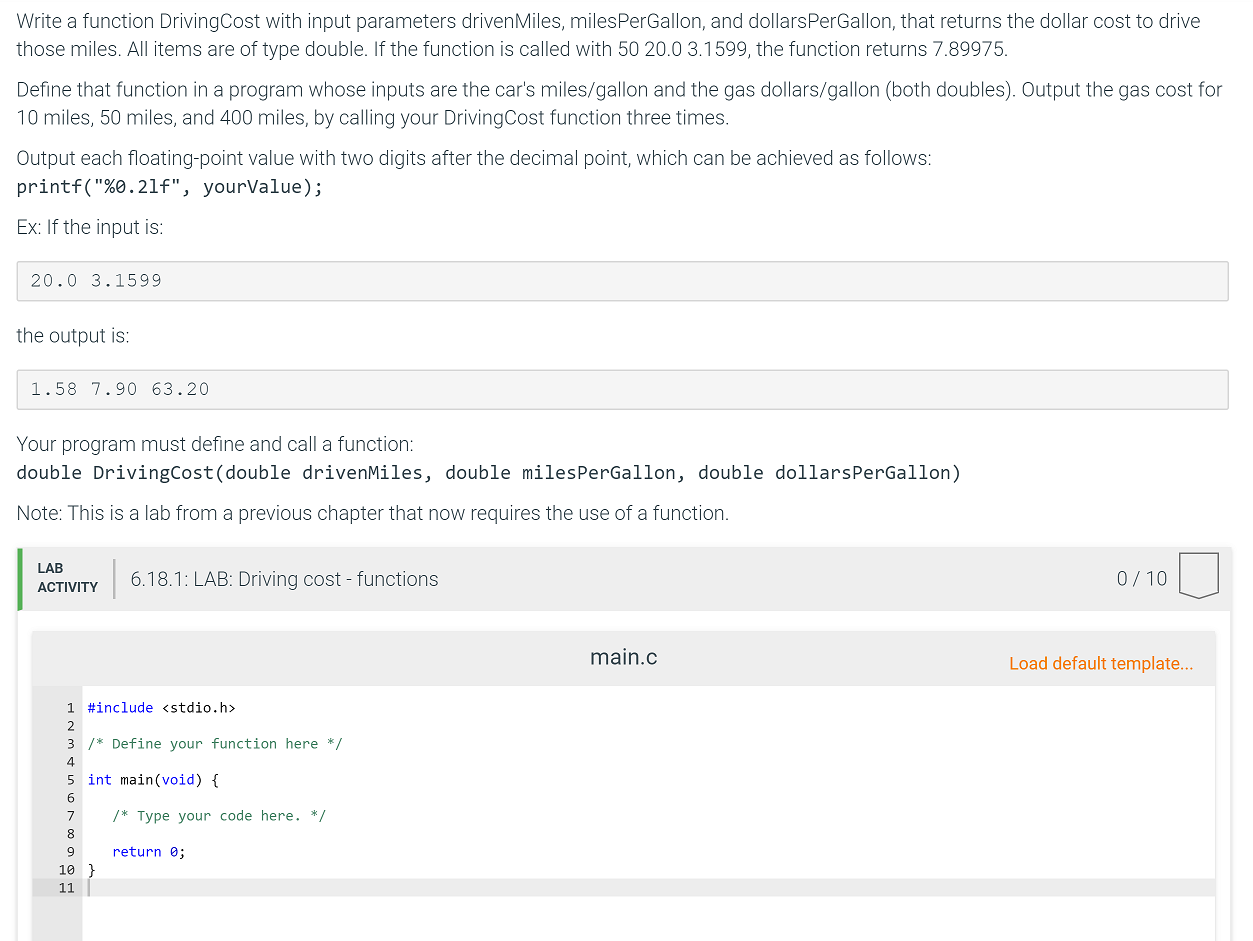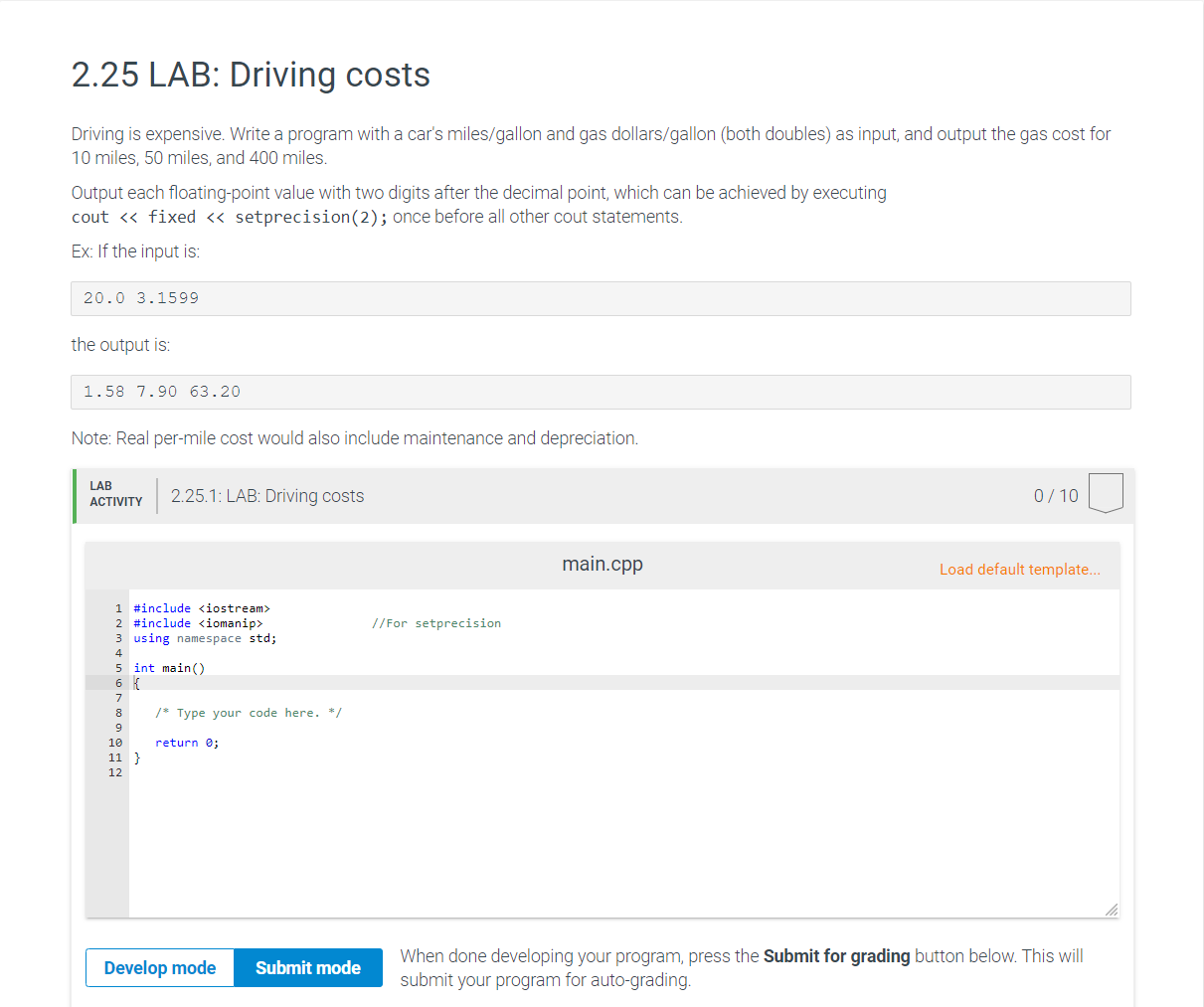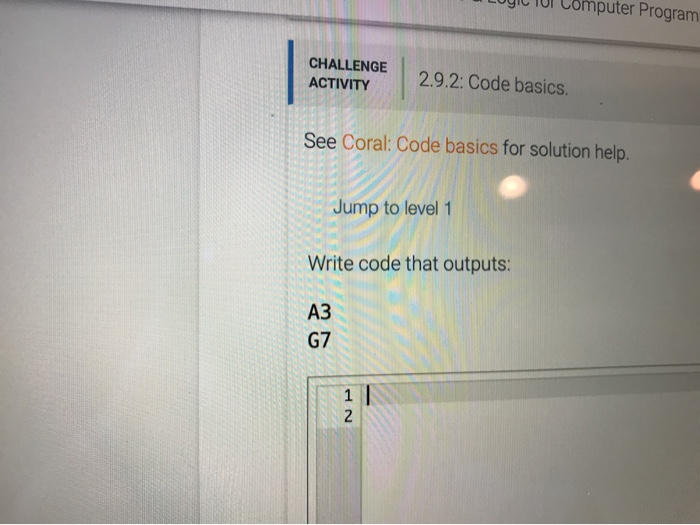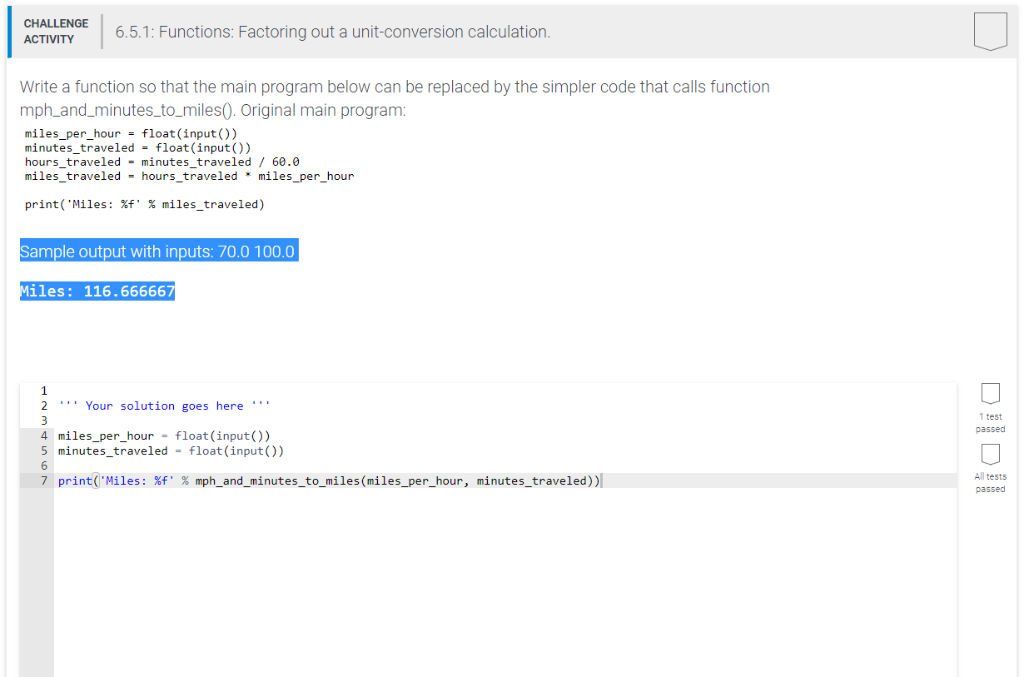Question

# Python 3 please. 6.27 (Functions) HW: Driving cost - functions Write a function DrivingCost with input...

6.27 (Functions) HW: Driving cost - functions

Write a function DrivingCost with input parameters drivenMiles, milesPerGallon, and dollarsPerGallon, that returns the dollar cost to drive those miles.

Ex: If the function is called with 50 20.0 3.1599, the function returns 7.89975.

Define that function in a program whose inputs are the car's miles/gallon and the gas dollars/gallon (both floats). Output the gas cost for 10 miles, 50 miles, and 400 miles, by calling your DrivingCost function three times.

Ex: If the input is:

```20.0
3.1599
```

Then the output is:

```1.57995
7.89975
63.198
```

Your program must define and call the following function:
def DrivingCost(drivenMiles, milesPerGallon, dollarsPerGallon)

Note: This is a lab from a previous chapter that now requires the use of a function.

```def DrivingCost(drivenMiles, milesPerGallon, dollarsPerGallon):
return drivenMiles / milesPerGallon * dollarsPerGallon

if __name__ == '__main__':
milesPerGallon = float(input())
dollarsPerGallon = float(input())
print(DrivingCost(10, milesPerGallon, dollarsPerGallon))
print(DrivingCost(50, milesPerGallon, dollarsPerGallon))
print(DrivingCost(400, milesPerGallon, dollarsPerGallon))
```

#### Earn Coins

Coins can be redeemed for fabulous gifts.

Similar Homework Help Questions
• ### Driving cost - methods

3.14 LAB: Driving cost - methodsWrite a method drivingCost() with input parameters drivenMiles, milesPerGallon, and dollarsPerGallon, that returns the dollar cost to drive those miles. All items are of type double. If the method is called with 50 20.0 3.1599, the method returns 7.89975.Define that method in a program whose inputs are the car's miles/gallon and the gas dollars/gallon (both doubles). Output the gas cost for 10 miles, 50 miles, and 400 miles, by calling your drivingCost() method three times.Output each...

• ### Write a method drivingCost() with input parameters drivenMiles, milesPerGallon, and dollarsPerGallo

6.26 LAB: Driving cost - methodsWrite a method drivingCost() with input parameters drivenMiles, milesPerGallon, and dollarsPerGallon, that returns the dollar cost to drive those miles. All items are of type double. If the method is called with 50 20.0 3.1599, the method returns 7.89975.Define that method in a program whose inputs are the car's miles/gallon and the gas dollars/gallon (both doubles). Output the gas cost for 10 miles, 50 miles, and 400 miles, by calling your drivingCost() method three times.Output...

• ### Write a function DrivingCost with input parameters drivenMiles, milesPerGallon, and dollarsPerGallonWrite a function DrivingCost with input parameters drivenMiles, milesPerGallon, and dollarsPerGallon, that returns the dollar cost to drive those miles. All items are of type double. If the function is called with 5020.03 .1599, the function returns 7.89975 .Define that function in a program whose inputs are the car's miles/gallon and the gas dollars/gallon (both doubles). Output the gas cost for 10 miles, 50 miles, and 400 miles, by calling your DrivingCost function three times.Output each floating-point value with two...

• ### PLEASE WRITE IN C++ 2.25 LAB: Driving costs Driving is expensive. Write a program with a...PLEASE WRITE IN C++ 2.25 LAB: Driving costs Driving is expensive. Write a program with a car's miles/gallon and gas dollars/gallon (both doubles) as input, and output the gas cost for 10 miles, 50 miles, and 400 miles. Output each floating-point value with two digits after the decimal point, which can be achieved by executing cout << fixed << setprecision(2); once before all other cout statements. Ex: If the input is: 20.0 3.1599 the output is: 1.58 7.90 63.20 Note:...

• ### LOGIC TUI Computer Program CHALLENGE ACTIVITY 2.9.2: Code basics See Coral: Code basics for solution help....LOGIC TUI Computer Program CHALLENGE ACTIVITY 2.9.2: Code basics See Coral: Code basics for solution help. Jump to level 1 Write code that outputs: A3 G7 TOUS: Home 3.6. Variables/Assignments: Driving costs My library > PRG 211: Algorithms & Logic for Computer Programming home > 6: Variables/Assignments: Driving costs zyBooks catalog He Start Driving is expensive. Write a program with a car's miles/gallon and gas dollars/gallon (both floats) as input, and output the gas cost for 10 miles, 50 miles,...

• ### Python Programing : Convert to binary - functions Write a program that takes in a positive...

Python Programing : Convert to binary - functions Write a program that takes in a positive integer as input, and outputs a string of 1's and 0's representing the integer in binary. For an integer x, the algorithm is: As long as x is greater than 0 Output x % 2 (remainder is either 0 or 1) x = x // 2 Note: The above algorithm outputs the 0's and 1's in reverse order. You will need to write a...

• ### 6.5.1: Functions: Factoring out a unit-conversion calculation. PYTHON CODING Write a function so that the main program b...6.5.1: Functions: Factoring out a unit-conversion calculation.PYTHON CODINGWrite a function so that the main program below can be replaced by the simpler code that calls function mph_and_minutes_to_miles(). Original main program:miles_per_hour = float(input()) minutes_traveled = float(input()) hours_traveled = minutes_traveled / 60.0 miles_traveled = hours_traveled * miles_per_hour print('Miles: %f' % miles_traveled)Sample output with inputs: 70.0 100.0Miles: 116.666667

• ### Write a program that first gets a list of integers from input. The input begins with...

Write a program that first gets a list of integers from input. The input begins with an integer indicating the number of integers that follow. Then, get the last value from the input, and output all integers less than or equal to that value. Ex: If the input is: 5 50 60 140 200 75 100 the output is: 50 60 75 The 5 indicates that there are five integers in the list, namely 50, 60, 140, 200, and 75....

• ### Objectives Work with functions Assignment Write each of the following functions using Python. The function header MUST b...

Objectives Work with functions Assignment Write each of the following functions using Python. The function header MUST be written as specified. In your main code test all of the specified functions. Each function must have a comment block explaining what it does, what the parameters are and what the return value is. Please remember the following two guidelines: unless the purpose of the function is to generate output DO NOT write to the screen within the function unless the purpose...

• ### Write a complete program that uses the functions listed below. Except for the printOdd function, main...

Write a complete program that uses the functions listed below. Except for the printOdd function, main should print the results after each function call to a file. Be sure to declare all necessary variables to properly call each function. Pay attention to the order of your function calls. Be sure to read in data from the input file. Using the input file provided, run your program to generate an output file. Upload the output file your program generates. •Write a...